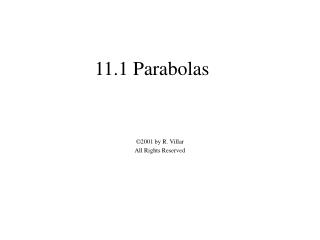DownloadDownload Presentation11.1 Parabolas

# 11.1 Parabolas

Télécharger la présentation## 11.1 Parabolas

- - - - - - - - - - - - - - - - - - - - - - - - - - - E N D - - - - - - - - - - - - - - - - - - - - - - - - - - -
##### Presentation Transcript

2. Parabolas Parabola: the set of points in a plane that are the same distance from a given point called the focus and a given line called the directrix. The cross section of a headlight is an example of a parabola... The light source is the Focus Directrix

3. Here are some other applications of the parabola...

4. d2 d1 Focus d2 d3 d1 d3 Vertex Directrix Notice that the vertex is located at the midpoint between the focus and the directrix... Also, notice that the distance from the focus to any point on the parabola is equal to the distance from that point to the directrix... We can determine the coordinates of the focus, and the equation of the directrix, given the equation of the parabola....

5. Standard Equation of a Parabola:(Vertex at the origin) (0, p) y = –p (If the x term is squared, the parabola is up or down) Equation Focus Directrix y2 = 4px (p, 0) x = –p Equation Focus Directrix x2 = 4py (If the y term is squared, the parabola is left or right)

6. Examples: Determine the focus and directrix of the parabola y = 4x2 : Since x is squared, the parabola goes up or down… Solve for x2 x2 = 4py y = 4x2 4 4 x2 = 1/4y Solve for p 4p = 1/4 p = 1/16 Focus: (0, p) Directrix: y = –p Focus: (0, 1/16) Directrix: y = –1/16 Let’s see what this parabola looks like...

7. Examples: Determine the focus and directrix of the parabola –3y2 – 12x = 0 : Since y is squared, the parabola goes left or right… Solve for y2 y2 = 4px –3y2 = 12x –3y2 = 12x –3 –3 y2 = –4x Solve for p 4p = –4 p = –1 Focus: (p, 0) Directrix: x = –p Focus: (–1, 0) Directrix: x = 1 Let’s see what this parabola looks like...

8. Examples: Write the standard form of the equation of the parabola with focus at (0, 3) and vertex at the origin. Since the focus is on the y axis,(and vertex at the origin) the parabola goes up or down… x2 = 4py Since p = 3, the standard form of the equation is x2 = 12y Example: Write the standard form of the equation of the parabola with directrix x = –1 and vertex at the origin. Since the directrix is parallel to the y axis,(and vertex at the origin) the parabola goes left or right… y2 = 4px Since p = 1, the standard form of the equation is y2 = 4x